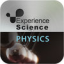Hlavní navigace

#Experience Physics 1.5

3

Pro hodnocení programu se prosím nejprve

Staženo
0 ×
Zdarma

## Sdílet

EXPERIENCE PHYSICS is a series of innovative apps designed to motivate students to investigate basic scientific phenomena and concepts in physics through simulations and interactive labs. Using an inquiry-based learning approach, the apps challenge students with investigations and quizzes based on the students' explorations of each interactive unit.

EXPERIENCE PHYSICS PROVIDES APPS WITH:

• Dynamic simulations of scientific phenomena and concepts, such as mass and weight, the relationship between distance, time and speed, and energy transformations between different types of energy;
• Multiple representations, such as dynamic graphs, tables, and interactive animations;
• Investigations that help students learn by exploring inquiry questions;
• Thought-provoking quizzes that are ideal for individual learning, small-group work, and teacher-led class instruction;
• Scientific background of the relevant concepts introduced in each unit.

EXPERIENCE PHYSICS UNITS INCLUDE:

Mass and Weight enables students to measure the mass and weight of different objects on Earth, Moon, Mars, and Jupiter using a balance scale (for mass) and a spring scale (for weight). Students can investigate the difference between mass and weight by using the simulation to answer these inquiry questions:
Is the mass of an object the same on different planets? Is the weight of an object the same on different planets? Based on your findings, what is the difference between mass and weight?

SPEED IT UP enables students to explore different speeds of a vehicle, based on distance-time graphs that describe its movement. Students can investigate the relationship between distance, time and speed by using the simulation to answer these inquiry questions:
How do different distance-time graphs describe different speeds? What happens to the slope of the graph when the speed of a vehicle changes? How does the graph describe one constant speed? How does it describe different constant speeds?

ENERGY OF A PENDULUM enables students to explore energy transformations of a swinging pendulum, with and without friction. Students can investigate potential energy, kinetic energy, thermal energy, and energy transformations by using the simulation to answer these inquiry questions:
What is the relationship between the pendulum's maximum potential energy at the beginning of its movement and its kinetic energy throughout the movement? Is this relationship the same with and without friction? If not, how does the relationship differ?

ENERGY OF A SKATEBOARD RIDER enables students to explore energy transformations of a skateboard and rider, with and without friction. Students can investigate potential energy, kinetic energy, thermal energy, and energy transformations by using the simulation to answer these inquiry questions:
What is the relationship between the skateboard's maximum potential energy at the beginning of its movement and its kinetic energy throughout the movement? Is this relationship the same with and without friction? If not, how does the relationship differ?

ENERGY OF AN ELASTIC SPRING enables students to explore energy transformations of an elastic spring, with and without friction. Students can investigate potential energy, kinetic energy, elastic energy, thermal energy, and energy transformations by using the simulation to answer these inquiry questions:
Assuming there is no friction in the system, what are the respective locations in the cycle at which the spring's potential, kinetic and elastic energies reach maximum values? What happens if you add friction to the system?

STOPPING DISTANCE invites students to conduct experiments that investigate the effects of vehicle speed and driver reaction time on the vehicle's stopping distance.

How does the driver's reaction time (slow or fast) affect the vehicle's stopping distance? How does the vehicle's speed affect its reaction distance and braking distance?

1.5

7. 3. 2017

0 ×

43,84 MB

Ano

• #### Podporované jazyky

• Angličtina
• ,
• Němčina
• ,
• Španělština
Zobrazit více
3

Pro hodnocení programu se prosím nejprve

Staženo
0 ×